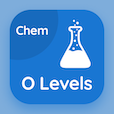Cambridge Online Courses (GCSE)

O Level Chemistry Quizzes

O Level Chemistry Quiz PDF - Complete

# Chemical and Ionic Equations Quiz MCQ Online p. 141

Practice Chemical and Ionic Equations quiz questions and answers PDF, chemical and ionic equations trivia questions 141 to learn online O Level Chemistry course for online classes. Chemical Formulae and Equations MCQ questions, chemical and ionic equations Multiple Choice Questions (MCQ) for online college degrees. "Chemical and Ionic Equations Quiz" PDF eBook: electrolyte and non electrolyte, endothermic reactions, chemical and ionic equations test prep for ACT subject test tutoring.

"To balance Cu + H2SO4 → CuSO4 + H2O + SO2; number of H2SO4 molecules will be" MCQ PDF: 2, 1, 3, and 4 for GRE practice test. Solve chemical formulae and equations questions and answers to improve problem solving skills for best online SAT prep class.

## Trivia Quiz on Chemical & Ionic Equations MCQs

MCQ: To balance Cu + H2SO4 → CuSO4 + H2O + SO2; number of H2SO4 molecules will be

1
2
3
4

MCQ: What is correct about burning of hydrogen?

it is an endothermic reaction
it acts as fuel in rockets
it is fatal for plant habitats
it results in acid rains

MCQ: In electrolysis of brine, ions moving to cathode include

sodium + hydrogen ions
hydroxide ions
chloride ions
hydrogen gas is bubbled off

MCQ: Nitric Acid (HNO3) manufacturing requires catalyst of

iron
platinum
nickel

MCQ: Conversion of solid Ammonium Nitrate (NH4NO3(s)) into aqueous Ammonium Nitrate (NH4NO3(aq)) is an example of

combustion
Thermal decomposition
Neutralization
dissolving

### More Quizzes from O Level Chemistry Course